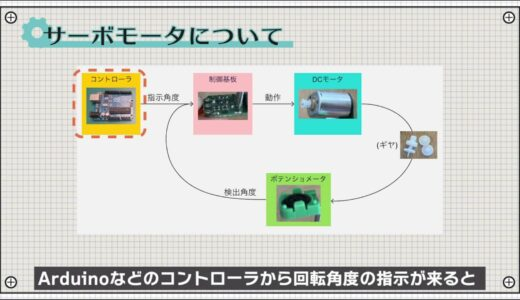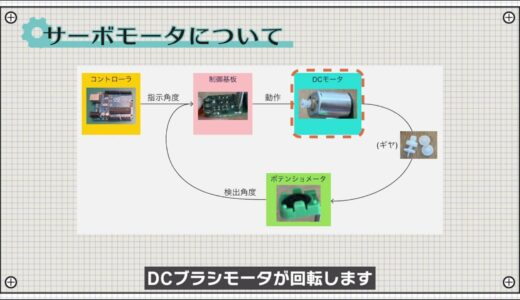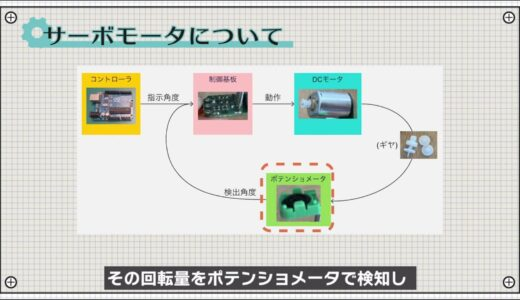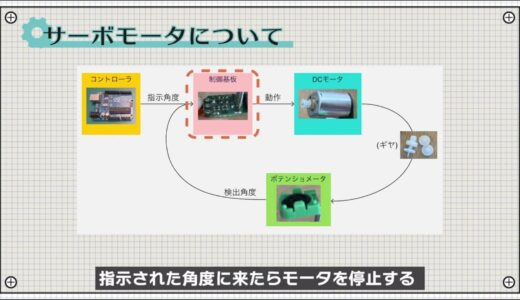# Arduino for Beginners】7.Servo Motor｜Hands-On Introductory Course for BeginnersSHARE

0:51 What we will make this time
0:58 Preparation of materials to be used
1:03 Schematic
1:27 Assembling the circuit
1:55 Programming
4:07 Write on the Board
4:30 Summary.

## 7. Servo motor

I'm new to electronics, but I' m stumped because I'm not sure about the repetition syntax.Then let's learn together about repetitive constructions in this course!

In this lesson, you will learn to use servo motors to

What you will learn in this lesson
• Repetition syntax: for
• Serial monitor (graph)

In particular, be sure to keep the for statement in mind, as it is a highly versatile program that will always appear in whatever you do.

## Codes used

This is the code used in the video. Please feel free to use it.

```//Import library for servo motor control
#include

// Create a servo motor object
Servo myservo;.

void setup() {
myservo.attach(9);
Serial.begin(9600);
}

void loop() {
//Rotate by 1 degree from 0 to 180 degrees
for (int angle = 0; angle <= 180; angle++) { myservo.write(angle); delay(30); Serial.println(angle); } delay(1000); }  ```

Servo motors are motors that can control the angle of rotation and speed, and are widely used when you want to move an object precisely within a certain angular range, such as joints in a robot or the opening and closing of a railroad crossing.As shown below, the structure consists of an ordinary brushed DC motor, some gears, a control board, and a potentiometer that detects the amount of rotation.Here is a simple system of servo motors!When the rotation angle instruction comes from a controller such as Arduino, the DC brush motor rotates.The amount of rotation is detected by a potentiometer, and the motor is stopped when it reaches the indicated angle.Such a system that detects the amount of change and determines the next action is generally called feedback control.

### What we will make this time

The following image shows the circuit we created this time, which operates to rotate 180 degrees every 5 seconds.Now let's make this circuit.

### Preparation of materials to be used

The materials used for this project are an Arduino Uno, a servo motor, and three male and three female jumper wires.The following image shows the schematic to be created for this project.Power for the servo motor is supplied from the 5V output terminal of the Arduino.In the case of the Arduino, the power supply for the 5V output terminal is directly connected to the USB port, allowing current to flow up to 500mA, the USB standard.
Large motors cannot be moved, but small servo motors for hobby use, such as the one used in this case, can be used without problems.

### schematic

Next, following the schematic, the breadboard diagram is shown below.Most servo motors, including the type used in this project, have three wires: orange, red, and brown.In this case, orange represents the signal, red the power supply, and brown the GND line, so do not make a mistake.### construct a circuit

Here is a circuit I built according to this diagram.### Programming

Now we will proceed with the coding.Start the Arduino IDE, click File→New File, and enter the program on this screen.Here we start with the first line of code called " include" code.and imports a library for servo motor control called Servo.h.

What is a library?
A collection of code prepared to operate a certain module.Arduino comes with several libraries by default, including this Servo.h.Utilizing libraries is easier than writing code from scratch, so be proactive about using them!

The servo.h used in this project has the following six functions, so please take a quick look at them before use.Then, in order to be able to use the library read out, an object is created in the following part as a preliminary step.An object is a bit difficult to conceptualize, but it is something that is called out from its blueprint state into the real world in order to actually be able to use its function.In the Setup function, the function attach in the servo library is used to assign pin 9 to a pin for a signal.Also, this time, to monitor values using a function called serial plotter, the Serial.begin function is used as well as the serial monitor function.Next, within the loop function, a process is written that repeats itself using a for statement.In a for statement, the program works in such a way that the portion enclosed within the braces is repeated as long as the condition in {} is satisfied.｛Inside {} are three sections: initialization process, conditional expressions, and increments.There are several ways to write increments, but keep in mind that they all mean the same thing!In this case, the variable angle, which represents the angle of rotation of the servo motor, is first assigned 0, and the process is repeated, adding 1 at a time, until 180 is reached.And in that part of the process, the function "write" in the servo library is used to rotate the angle to be the angle of each ANGLE.It then waits for a period of 30 ms and also allows the current value of angle to be viewed on a serial plotter.This simply means rotating from 0 to 180 degrees in 1 degree increments.And the rotation time is 30 ms per degree, which means about 5 seconds at 180 degrees.

After rotating to 180 degrees, wait 1 second and repeat the same operation from 0 degrees again.### Write on board

Finally, save the file and press the "Write to microcontroller board" button on the toolbar to write the file.If the program was created without any problems, you would see a 180-degree rotation every 5 seconds.Also, if you start the serial plotter from the tool on the menu bar, you will see that it gradually increases from 0 to 180, and when it reaches 180, it starts from 0 again, as shown in the following image.## summary

So this is what we have learned in this issue.

What I learned from this lesson
• Repeated syntax; for